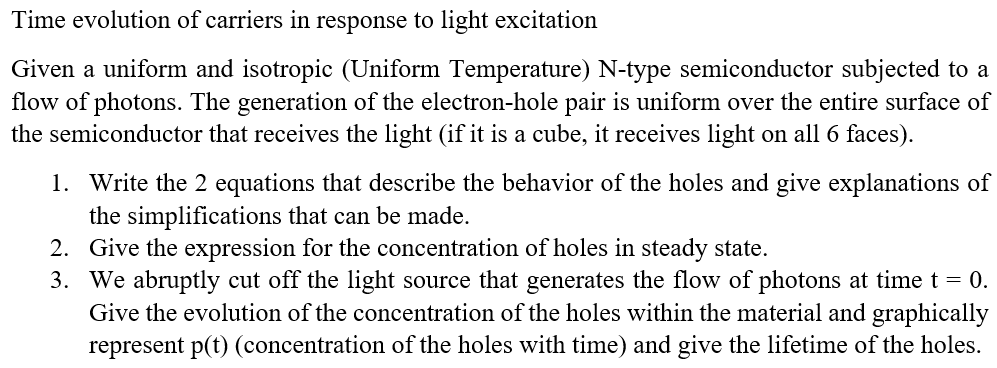Home / Expert Answers / Electrical Engineering / time-evolution-of-carriers-in-response-to-light-excitation-given-a-uniform-an-pa937

# (Solved): Time evolution of carriers in response to light excitation Given a uniform an ...???????

Time evolution of carriers in response to light excitation Given a uniform and isotropic (Uniform Temperature) N-type semiconductor subjected to a flow of photons. The generation of the electron-hole pair is uniform over the entire surface of the semiconductor that receives the light (if it is a cube, it receives light on all 6 faces). 1. Write the 2 equations that describe the behavior of the holes and give explanations of the simplifications that can be made. 2. Give the expression for the concentration of holes in steady state. 3. We abruptly cut off the light source that generates the flow of photons at time $$t=0$$. Give the evolution of the concentration of the holes within the material and graphically represent $$\mathrm{p}(\mathrm{t})$$ (concentration of the holes with time) and give the lifetime of the holes.

We have an Answer from Expert